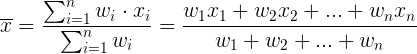# Weighted Average Calculator

Weighted average calculator and calculation. Weighted mean calculator.

 Weight Data number Weighted average: Total of weights: Calculation:

Average calculator ►

## Weighted average calculation

The weighted average (x) is equal to the sum of the product of the weight (wi) times the data number (xi) divided by the sum of the weights:### Example

Find the weighted average of class grades (with equal weight) 70,70,80,80,80,90:

Since the weight of all grades are equal, we can calculate these grades with simple average or we can cound how many times each grade apear and use weighted average.

2×70,3×80,1×90

x = (2×70+3×80+1×90) / (2+3+1) = 470 / 6 = 78.33333